#20 Simple Interest || Learn Python Foundation in Tamil

Next topic: #21 Compound Interest : https://www.youtube.com/watch?v=12TdKUbqWHA&list=PLmjuBlzAWCzy3DO_bSzaUcNLLgE-Xtnai&index=21

This Video explains how to solve this problem in python.

https://tinyurl.com/in-collectiva-ckaapp

9 thoughts on “#20 Simple Interest || Learn Python Foundation in Tamil”

1.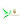srinivas s says:

Sir , if i give two input () . It doesn't wait for my second input. i am using pycharm. If i give single input it is working. I don't it was a coding issue. Can you please help me to fix it.
my code is,

# Accepting the input

Pri = float(input("Enter the Princpal Amount: "))

Num = float(input("Enter the No of years: "))

Rate = float(input("Enter the Rate of Interest: "))

# Calculate the Simple Interest

SI = (Pri * Num * Rate) / float(100)

# Output the calculated Simple Interest

print("Simple Interest is:", SI)

Error #:
Enter the Princpal Amount:2300

Enter the No of years: Traceback (most recent call last):

File "G:/python projects/simple/simple programs/simpleinterest.py", line 4, in <module>

Num = float(input("Enter the No of years: "))

ValueError: could not convert string to float:

Process finished with exit code 1

2.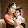Mani M.V says:

Sir I'm getting error like::
Can't multiply sequence by non-int of type 'str'

I think, we will get the above error in Python 3 , if we execute the same SimpleInterest program. So it should be modified like as shown below, Is it correct?

# 21.11.2018
# Chapter 20 : Simple Interest program

# Accepting the input
Pri = float(input("Enter the Princpal Amount: "))
Num = float(input("Enter the No of years: "))
Rate = float(input("Enter the Rate of Interest: "))

# Calculate the Simple Interest
SI = (Pri * Num * Rate) / float(100)

# Output the calculated Simple Interest
print("Simple Interest is:", SI)
print("Simple Interest is: {}".format(SI))
print("Simple Interest is: {:.2f}".format(SI))

3.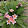AGALYA DEVI says:

Not clear view
It's blurred

4.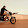Kamal 08 says:

Sir line 12 a marupadium explain panna nallairukkum!

5.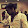Sakthivel Murugan says:

Sir I'm getting error like::
Can't multiply sequence by non-int of type 'str'

6.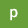parthi ban says:

Can I modify the last line as simple as like below

print "the simple interest is %.2f" %(simpleinterest)

will it create any problem? please comment.

7.nancy anto says:

how to take 2f rate of interst

8.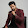tech trends says:

sir , na kali linux os use panran,itha program ah terminal la use panna out correct ah varuthu but pycharm use pana error varuthu

9.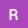Ramya R says:

sir why we are using .2f